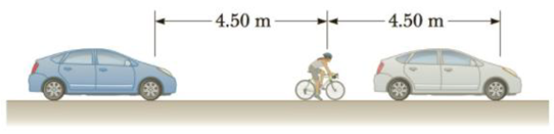Chapter 14, Problem 37P

Chapter
Section
Textbook Problem

Two cars are stuck in a traffic jam and each sounds its horn at a frequency of 625 Hz. A bicyclist between the two cars, 4.50 m from each horn (Fig. P14.37), is disturbed to find she is at a point of constructive interference. How far backward must she move to reach the nearest point of destructive interference?Figure P14.37

To determine
Distance to reach the nearest point of destructive interference.

Explanation

Given Info: Frequency of sound from the horn is 625Hz, the distance of bicyclist from each horn is 4.50 m.

Formula for wavelength,

λ=vf

• v is the speed of the sound,
• f is the frequency of  sound from horn,

For destructive interference the path difference is half integral multiple of wavelength. So, the nearest point of destructive interference occurs at λ/2 . That is, when path difference between the two sound waves reaching the bicyclist is equal to the λ/2 .

Formula for total distance to nearest destructive interference,

(dcar+d)(dcard)=λ2

• dcar is the distance of car from the bicyclist,
• d is the nearest point of destructive interference,

Use v/f for λ in the above expression to rewrite it.

(dcar+d)(dcard)=v2f

Substitute 343m/s for v, 625Hz for f , and 4

Still sussing out bartleby?

Check out a sample textbook solution.

See a sample solution

The Solution to Your Study Problems

Bartleby provides explanations to thousands of textbook problems written by our experts, many with advanced degrees!

Get Started